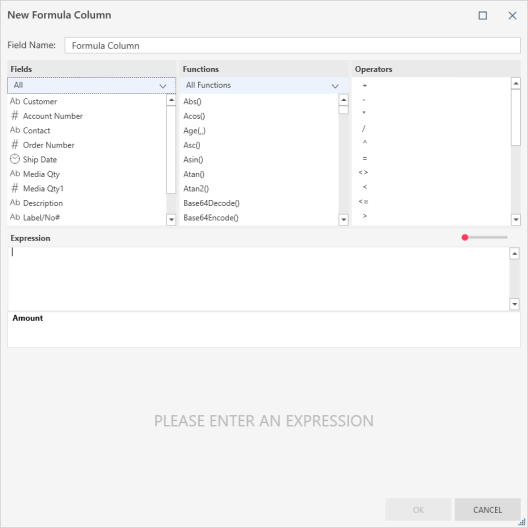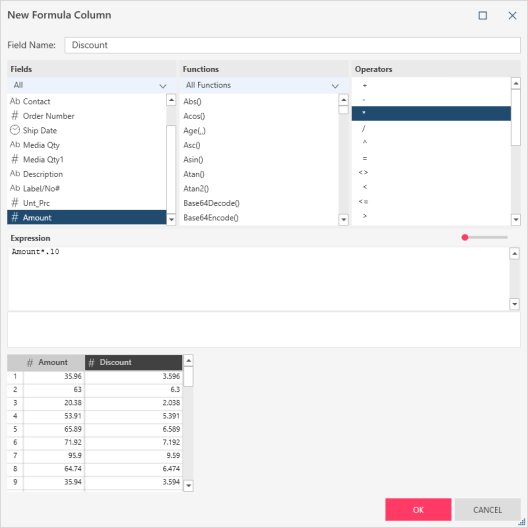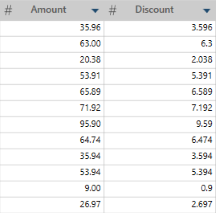# Creating New Formula Columns

### Description

Creates a new column based on a calculation.

1. Right+Click on a column and select Column > New Formula Column from the options that display. The New Formula Column dialog displays:2. Rename this column by replacing the name indicated in the Field Name field.

3. Define the expression for calculating the new field value:

Note that:

• Select fields, operators, functions, and operators from the lists above the expression builder.

• Refer to functions reference for a list and description of all functions you can use within an expression.

•• You can use the drop-down provided below the Functions column to specify a set of functions to display (e.g., Date, String, Numeric, Trigonometry, etc.).

• As you define the formula, a sample of the output is displayed in the preview area at the bottom of the dialog box.

• An automatic suggestion popup displays when you type into the Expression box. The suggestions include the functions and all the field names. When selected, the suggestion will be inserted into the formula in the same manner as double-clicking an option from the lists above the formula box.4. Select OK to add the specified calculation as a new field to your table.

### Example

In this example, the first column (Amount) is the original column. Discount is a calculated field that shows the 10% discount value. It uses the following simple formula: Amount * .10:Other expressions can look like this:

• If(Customer="Ace Resellers",Unit_price*.55, Unit_price*.65)

• FiscalWeek([Ship Date])

• Replace(Contact,"Smith","Smith-Jones")